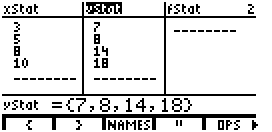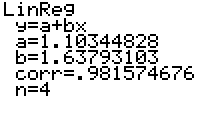# Knowledge Base

## Solution 10414: Calculating Linear Regressions on the TI-86.

### How do I calculate a linear regression on the TI-86?

The example below will demonstrate how to calculate a linear regression on the TI-86.

Data for this example:
xstat(3,5,8,10)
ystat(7,8,14,18)

Enter the data into the TI-86:

• Enter STAT mode by pressing [2nd] [STAT].
• Press [F2] to select Edit.
• Enter the data in the xStat and yStat lists.
• Press [2nd] [QUIT] to leave the editor.To calculate the Linear Regression (a+bx):

• Enter the STAT mode again by pressing [2nd] [STAT].
• Press [F1] to select CALC.
• Press [F3] to select LinR.
• Now press [2nd] [LIST] to select the lists to use for the regression.
• Press [F3] to select Names. Press the function key (F1-F5) that corresponds to xStat, press the comma button, then select yStat.
• Press [ENTER] to perform the regression calculation.The calculator will display the form of the equation (y=a+bx) and then list the values for the two coefficients (a and b). It will also list a value for corr. This is a correlation coefficient, and it needs to be as close as possible to 1 to ensure a good curve fit. 1.0 is a perfect fit, and anything less is an approximation. For example, .999 would be an excellent fit. The final value listed is the number of points that were analyzed to generate the equation.